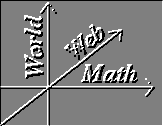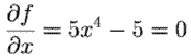#The Critical Point Test

Prequisites: Partial Derivatives

So, Say a point P0=(a1,a,2,...an ,) is a maximum point (or a minimum pont), of the equation y= f(x1,x,2,...xn,) . Then if we hold (x2,...xn) fixed at (a2,...an) , f becomes a function of x1 only, with a maximum at x1=a1.

Therefore at this point.

Likewise, fx2=0, fx3=0, ... fxn=0 at point P.

A Critical Point is any point that satisfies these n equations. Alternately, the critical point is any point on our n-dimensional surface whose tangential n-1 surface is parallel to the x1-axis, x2-axis, ... , and xn-axis. We can determine critical points (a1,a2,... an) by solving these n equations for the n unknowns .

For example, say f(x,y)=x5+y4-5x-32y. Then,

##,.

Solving for real critical points, we get (1,2) and (-1,2). But are these points minimas or maxima, or neither? We notice that the point x=1 is a minimum for the first equation and the point y=2 is a minimum for the second equation. Therefore, the point (1,2) is a minimum for f(x,y)=x5+y4-5x-32y . On the other hand, the point x=-1 is a maximum for the first equation. Thus, this surface, viewed along the x-axis, comes to a maximum, while viewed along the y-axis, comes to a minimum. The critical point of (-1,2) is neither a minimum nor a maximum point for the surface. It is a saddle point .

Below are images of a minimum, a maximum, and a saddle point critical point for a two-variable function.

##In the next section we will deal with one method of figuring out whether a point is a minimum, maximum, or neither.

Vector Calculus Index | World Web Math Main Page

watko@athena.mit.edu